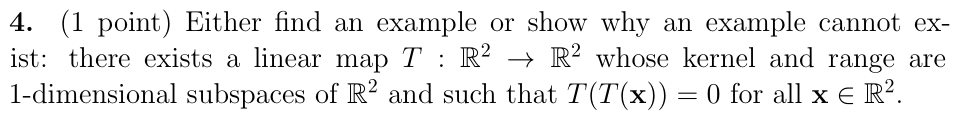Home / Answered Questions / Other / 4-1-point-either-find-an-example-or-show-why-an-example-cannot-ex-ist-there-exists-a-linear-map-t-r2-aw319

# (Solved): 4. (1 Point) Either Find An Example Or Show Why An Example Cannot Ex- Ist: There Exists A Linear Map...4. (1 point) Either find an example or show why an example cannot ex- ist: there exists a linear map T : R2 + R2 whose kernel and range are 1-dimensional subspaces of R2 and such that T(T(x)) = 0 for all x â‚¬ R2.

We have an Answer from Expert